# Griffiths Quantum Mechanics 2nd edition Chapter 8 equation is confusing

Homework Statement:
Griffiths Quantum Mechanics 2nd edition
Relevant Equations:
Griffiths second edition equations 8.24 and 8.25
In Griffiths Quantum Mechanics 2nd edition, in Chapter 8 he calculates the following integral on page 323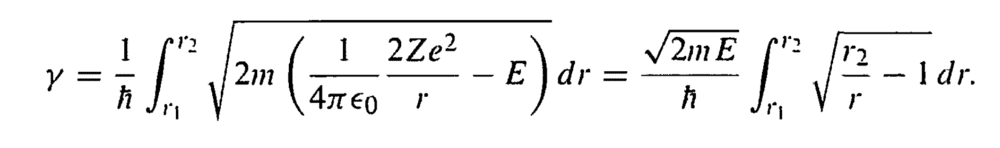and he gets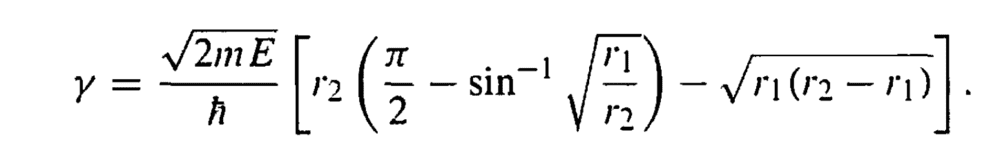I disagree with this result, I think the integral should be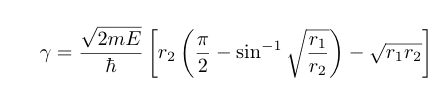since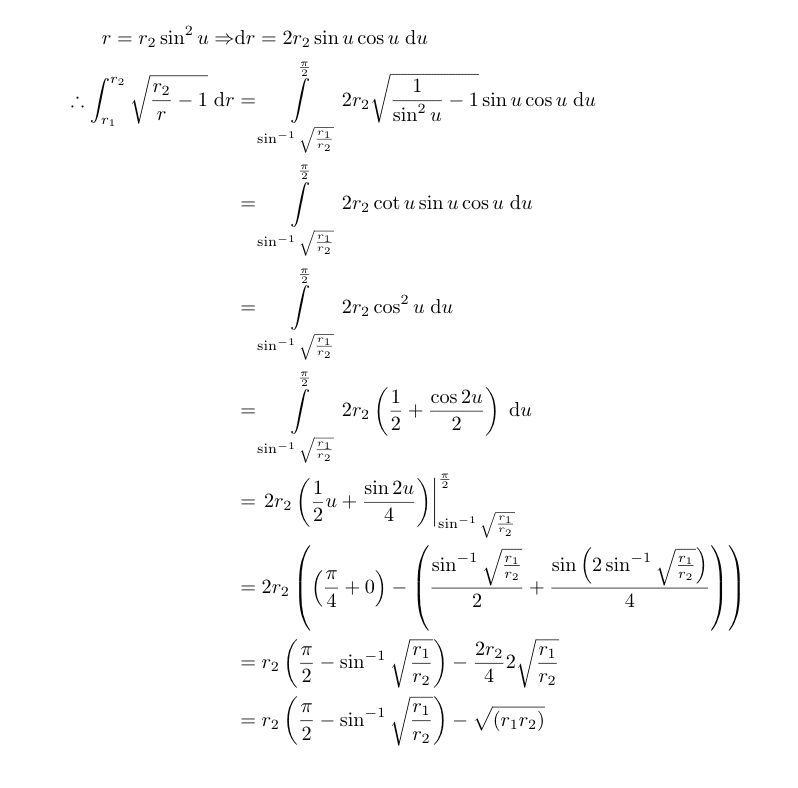Maybe somebody can explain why I am wrong? Also, from equation 8.24 to 8.25, he makes the assumption that sinϵ ≅ ϵ, but how does that imply sin^(-1)ϵ ≅ ϵ, which seems to be what he assumes to get from 8.24 to 8.25. Any insight is greatly appreciated!

#### Attachments

•Delta2

TSny
Homework Helper
Gold Member
I disagree with this result, I think the integral should be
View attachment 270765
Note that the integral must go to zero as ##r_2## approaches ##r_1##. But your result doesn't satisfy this condition.

Check the following: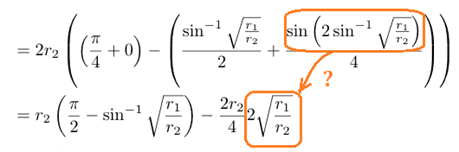Also, from equation 8.24 to 8.25, he makes the assumption that sinϵ ≅ ϵ, but how does that imply sin^(-1)ϵ ≅ ϵ, which seems to be what he assumes to get from 8.24 to 8.25. Any insight is greatly appreciated!
Take the inverse sine of both sides of sinϵ ≅ ϵ. Or, do a Taylor series of ##\sin^{-1} x## about ##x=0## .

•Delta2
Thank you, I get it now!

•berkeman, Delta2 and TSny